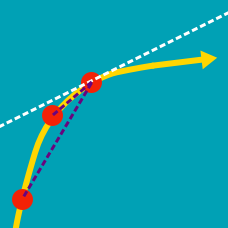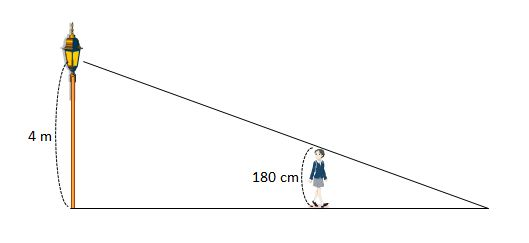Calculus

# Instantaneous Rate of Change

Let $f(x)=6 x^2+7x-5$. Then if the average rate of change of $f(x)$ when $x$ changes from $0$ to $18$ is the same as the rate of change of $f(x)$ at $x=a$, what is the value of $a$?

What is the rate of change of $y = \frac{x(x-5)^2}{(x+3)^3}$ at $x = 1$?

For a function $f(x)= x^2+px+q$, if the average rate of change of $f(x)$ when $x$ changes from $a$ to $b$ is the same as the rate of change at $x=c$, what is the value of $c$?

A person $180$ cm tall walks away from the base of a streetlight $4$ m high. If she follows a straight line at a velocity of $121$ m/min, what is the rate of change of the length of her shadow (in m/min)?What is the rate of change of the function $y = \ln ( 8 x)$ when $x = \frac{ 1}{16}$?

×